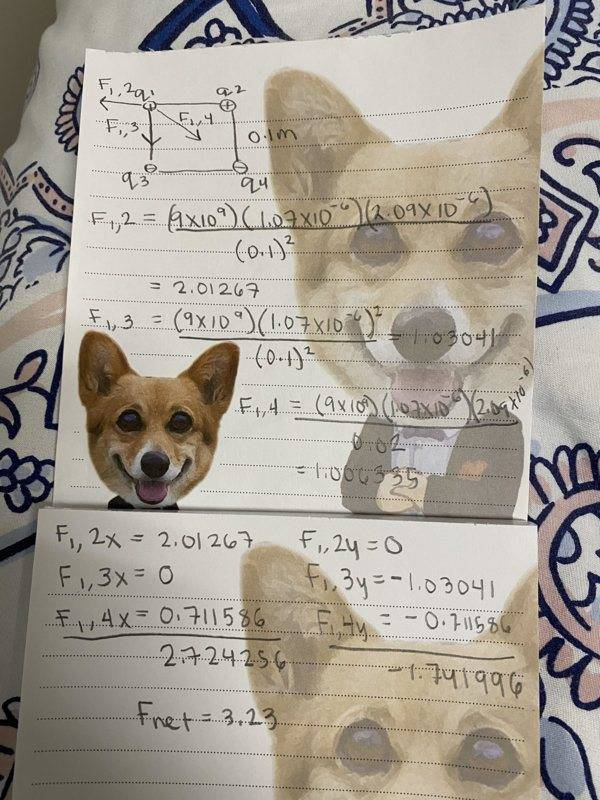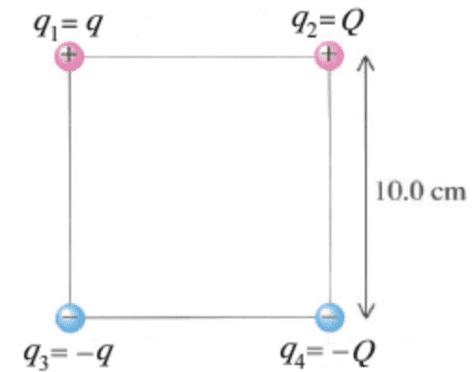# Coulomb's Law Problem

• moeug1999

#### moeug1999

Homework Statement
Consider the following figure. (The four charges are at the corners of a square. Take q = 1.07 µC and Q = 2.09 µC.)

(a) In the figure, what are the magnitude and direction of the resultant force on q1? (Take q1 as the origin of the coordinate system and measure the angle counterclockwise from the positive x-axis, which is directed towards the right.)

(b) What is the resultant force on the center of mass of the four charges?
Relevant Equations
The Coulombs law equation = k |q1||q2|/r^2This is my attempt but they said my answer is wrong

#### Attachments

Hello moeug ,!
moeug1999 said:
In the figure, what are the magnitude and direction of the resultant force on q1?
...
This is my attempt(the dog picture distracts from reading and checking the math)

(b) What is the resultant force on the center of mass of the four charges?
Is this serious ? Masses are not given and there is no question of a force out of the blue at any point. Was teacher in gross error here or is the problem statement misquoted ?

there are no masses given at all :(

BvU said:
Hello moeug ,!